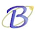Life is like riding a bicycle.  To keep your balance you must keep moving.”
Albert Einstein

Wednesday, May 30, 2012

AP Physics B & C - Multiple Choice Practice Questions on One Dimensional Kinematics

"Whenever you are confronted with an opponent, conquer him with love."
– Mahatma Gandhi

Let us discuss a few interesting multiple choice practice questions on one dimensional motion. Here are some questions beneficial for AP Physics B as well as AP Physics C aspirants:
(1) The adjoining figure shows the velocity time graph of an object. Total displacement suffered by the object during the interval when it has non-zero acceleration and retardation is
(a) 80 m
(b) 70 m
(c) 60 m
(d) 40 m
(e) 30 m
The object has non-zero acceleration and retardation during the time intervals from 5 sec to 15 sec and from 20 sec to 30 sec. The total area under the velocity time graph during these intervals gives the required displacement.
Displacement from 5 sec to 15 sec = 30 m
Displacement from 20 sec to 30 sec = 40 m
Therefore, total displacement = 70 m
(2) Successive positions (x) of an object (moving from left to right) at equal time intervals are shown in the following figure:

Which one among the following position-time graphs best represents the motion of the object?Change of position is slowest in the beginning and in the end. The motion is best represented by graph (c).
[Note that graph (d) is not the answer since the change of position in the beginning and in the end is shown as fastest in it]
(3) Which one among the following velocity-time graphs best represents the motion of the object mentioned in question no.(2)?

The graph (c) is the answer.
The following questions are meant for AP Physics C aspirants:
(4) A particle projected vertically upwards attains the maximum height h in time t. While returning from the highest point it takes an additional time t1 to fall to the height h.2. If air resistance is negligible, how is t1 related to t?
(a) t1 = t/2
(b) t1 = √(t/2)
(c) t1 = t/2
(d) t1 = t/3
(e) t1 = t/3
For the upward motion we have
0 – u2 = – 2gh …………(i)
[We have used the equation of motion, v2 u2 = 2as. The sign of the gravitational acceleration g is negative since it is opposite to the direction of the velocity of projection u]
Using the equation of motion, v = u + at we have
0 = u gt from which u = gt
Substituting this value of u in Eq (i), we have
g2t2 = 2gh
Therefore, t =√(2h/g) ……..(ii)
For the fall through h/2 from the highest point we have
h/2 = 0×t1 + ½ gt12
[We have used the equation of motion, s = ut + ½ gt2]
Therefore, t1 =√(h/g)
Comparing this with the value of t given in Eq (ii) we obtain t1 = t/2.
(5) A ball projected vertically up has the same vertical displacement h at times t1 second and t2 second. If air resistance is negligible, the maximum height reached by the ball is
(a) gt1t2/2
(b) g(t12 + t22)/2
(c) g(t1 + t2)2/4
(d) g(t1 + t2)2/8
(e) 2g(t1 + t2)2
The time taken by the ball to move from height h to the top of its trajectory and back to the height h is t2 t1. Therefore, the time taken to move from height h to the top of the trajectory is (t2 t1)/2.
The total time taken for the upward journey (from ground to the top most point) is evidently t1 + t2 t1)/2 = (t1 + t2)/2.
Time taken for the return journey (from the top most point to the ground) also is equal to (t1 + t2)/2. Therefore, the maximum height H (using the equation, s = ut + ½ gt2) is given by
H = 0 + ½ g [(t1 + t2)/2]2
Or, H = g(t1 + t2)2/8
Questions on kinematics were discussed earlier on this site. You can access them either by clicking on the label ‘kinematics’ below this post or by trying a search for                  ‘kinematics’ using the search box provided on this page.

1 comment:

1.